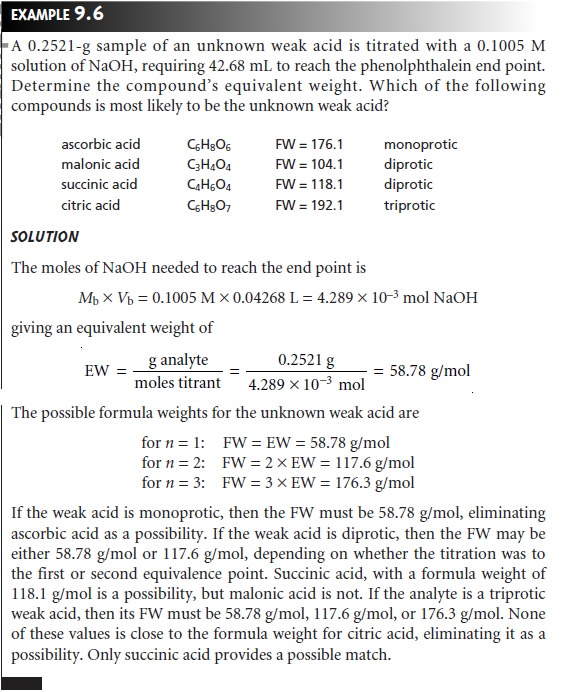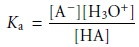Home | | Modern Analytical Chemistry | Titrations Based on Acidâ€“Base Reactions: Characterization Applications

# Titrations Based on Acidâ€“Base Reactions: Characterization Applications

Two useful characterization applications involving acidâ€“base titrimetry are the determination of equivalent weight, and the determination of acidâ€“base dissociation constants.

Characterization Applications

Two useful characterization applications involving acidâ€“base titrimetry are the determination of equivalent weight, and the determination of acidâ€“base dissociation constants.

## Equivalent Weights

Acidâ€“base titrations can be used to characterize the chemical and physical properties of matter. One simple example is the determination of the equivalent weight* of acids and bases. In this method, an accurately weighed sample of a pure acid or base is titrated to a well-defined equivalence point using a monoprotic strong acid or strong base. If we assume that the titration involves the transfer of n protons, then the moles of titrant needed to reach the equivalence point is given as

Moles titrant = n x moles analyte

and the formula weight isSince the actual number of protons transferred between the analyte and titrant is uncertain, we define the analyteâ€™s equivalent weight (EW) as the apparent formula weight when n = 1. The true formula weight, therefore, is an integer multiple of the calculated equivalent weight.

FW = n x EW

Thus, if we titrate a monoprotic weak acid with a strong base, the EW and FW are identical. If the weak acid is diprotic, however, and we titrate to its second equivalence point, the FW will be twice as large as the EW.## Equilibrium Constants

Another application of acidâ€“base titrimetry is the determi- nation of equilibrium constants. Consider, for example, the titration of a weak acid, HA, with a strong base. The dissociation constant for the weak acid isWhen the concentrations of HA and Aâ€“ are equal, equation 9.9 reduces to Ka = [H3O+], or pH = pKa. Thus, the pKa for a weak acid can be determined by measuring the pH for a solution in which half of the weak acid has been neutralized. On a titration curve, the point of half-neutralization is approximated by the volume of titrant that is half of that needed to reach the equivalence point. As shown in Figure 9.20, an estimate of the weak acidâ€™s pKa can be obtained directly from the titra- tion curve.This method provides a reasonable estimate of the pKa, provided that the weak acid is neither too strong nor too weak. These limitations are easily appreciated by considering two limiting cases. For the first case letâ€™s assume that the acid is strong enough that it is more than 50% dissociated before the titration begins. As a result the concentration of HA before the equivalence point is always less than the con- centration of Aâ€“, and there is no point along the titration curve where [HA] = [Aâ€“]. At the other extreme, if the acid is too weak, the equilibrium constant for the titra- tion reactionmay be so small that less than 50% of HA will have reacted at the equivalence point. In this case the concentration of HA before the equivalence point is always greater than that of Aâ€“.

Determining the pKa by the half-equivalence point method overes- timates its value if the acid is too strong and underestimates its value if the acid is too weak.

A second approach for determining the pKa of an acid is to replot the titration curve in a linear form as a Gran plot. For example, earlier we learned that the titra- tion of a weak acid with a strong base can be plotted in a linear form using the fol- lowing equationPlotting Vb x [H3O+] versus Vb, for volumes less than the equivalence point volume yields a straight line with a slope of â€“Ka. Other linearizations have been developed that use all the points on a titration curve or require no assumptions. This ap- proach to determining acidity constants has been used to study the acidâ€“base prop- erties of humic acids, which are naturally occurring, large-molecular-weight organic acids with multiple acidic sites. In one study, a sample of humic acid was found to have six titratable sites, three of which were identified as carboxylic acids, two of which were believed to be secondary or tertiary amines, and one of which was iden- tified as a phenolic group.

Study Material, Lecturing Notes, Assignment, Reference, Wiki description explanation, brief detail
Modern Analytical Chemistry: Titrimetric Methods of Analysis : Titrations Based on Acidâ€“Base Reactions: Characterization Applications |